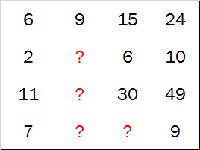BrainDen.com - Brain Teasers
• 0

# Find the missing numbers

## Question

Which are the missing numbers?(a)(b)(c)(d)## Recommended Posts

• 0
Spoiler

My answer is choice B. This is because the numbers in the grid have a pattern within the horizontal rows. This pattern is that the last two numbers in the row are equal to the previous two numbers added together.

6    9    15    24               6+9=15   9+15=24

2    4     6     10               2+4=6     4+6=10

11  19  30   49               11+19=30  19+30=49

7    1     8      9                7+1=8     1+8=9

This pattern works with answer choice B throughout the grid of numbers, so that is my final answer.

##### Share on other sites

• 0
On 10/31/2017 at 1:11 AM, Buddyboy3000 said:
Reveal hidden contents

My answer is choice B. This is because the numbers in the grid have a pattern within the horizontal rows. This pattern is that the last two numbers in the row are equal to the previous two numbers added together.

6    9    15    24               6+9=15   9+15=24

2    4     6     10               2+4=6     4+6=10

11  19  30   49               11+19=30  19+30=49

7    1     8      9                7+1=8     1+8=9

This pattern works with answer choice B throughout the grid of numbers, so that is my final answer.

Yeap, this is the correct answer!

## Join the conversation

You can post now and register later. If you have an account, sign in now to post with your account.×   Pasted as rich text.   Paste as plain text instead

Only 75 emoji are allowed.

×   Your previous content has been restored.   Clear editor

×   You cannot paste images directly. Upload or insert images from URL.